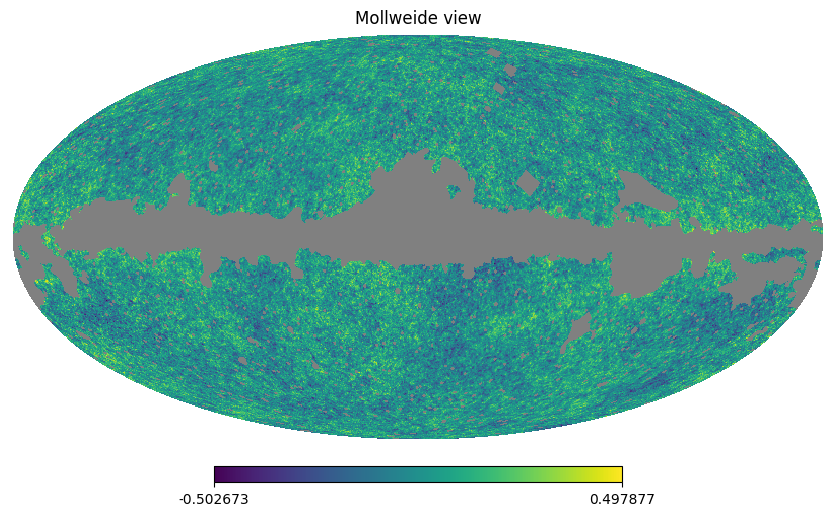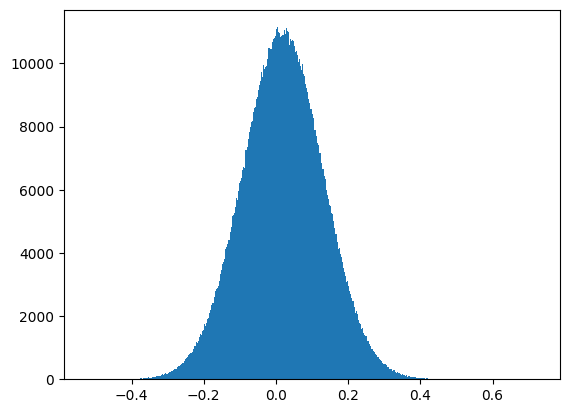# healpy tutorial¶

See the Jupyter Notebook version of this tutorial at https://github.com/healpy/healpy/blob/master/doc/tutorial.ipynb

Choose the inline backend of maptlotlib to display the plots inside the Jupyter Notebook

:

import matplotlib.pyplot as plt

%matplotlib inline

:

import numpy as np
import healpy as hp


## NSIDE and ordering¶

Maps are simply numpy arrays, where each array element refers to a location in the sky as defined by the Healpix pixelization schemes (see the healpix website).

Note: Running the code below in a regular Python session will not display the maps; it’s recommended to use an IPython shell or a Jupyter notebook.

The resolution of the map is defined by the NSIDE parameter, which is generally a power of 2.

:

NSIDE = 32
print(
"Approximate resolution at NSIDE {} is {:.2} deg".format(
NSIDE, hp.nside2resol(NSIDE, arcmin=True) / 60
)
)

Approximate resolution at NSIDE 32 is 1.8 deg


The function healpy.pixelfunc.nside2npix gives the number of pixels NPIX of the map:

:

NPIX = hp.nside2npix(NSIDE)
print(NPIX)

12288


The same pixels in the map can be ordered in 2 ways, either RING, where they are numbered in the array in horizontal rings starting from the North pole:

:

m = np.arange(NPIX)
hp.mollview(m, title="Mollview image RING")
hp.graticule()

0.0 180.0 -180.0 180.0The standard coordinates are the colatitude $$\theta$$, $$0$$ at the North Pole, $$\pi/2$$ at the equator and $$\pi$$ at the South Pole and the longitude $$\phi$$ between $$0$$ and $$2\pi$$ eastward, in a Mollview projection, $$\phi=0$$ is at the center and increases eastward toward the left of the map.

We can also use vectors to represent coordinates, for example vec is the normalized vector that points to $$\theta=\pi/2, \phi=3/4\pi$$:

:

vec = hp.ang2vec(np.pi / 2, np.pi * 3 / 4)
print(vec)

[-7.07106781e-01  7.07106781e-01  6.12323400e-17]


We can find the indices of all the pixels within $$10$$ degrees of that point and then change the value of the map at those indices:

:

ipix_disc = hp.query_disc(nside=32, vec=vec, radius=np.radians(10))

:

m = np.arange(NPIX)
m[ipix_disc] = m.max()
hp.mollview(m, title="Mollview image RING")We can retrieve colatitude and longitude of each pixel using pix2ang, in this case we notice that the first 4 pixels cover the North Pole with pixel centers just ~$$1.5$$ degrees South of the Pole all at the same latitude. The fifth pixel is already part of another ring of pixels.

:

theta, phi = np.degrees(hp.pix2ang(nside=32, ipix=[0, 1, 2, 3, 4]))

:

theta

:

array([1.46197116, 1.46197116, 1.46197116, 1.46197116, 2.92418036])

:

phi

:

array([ 45. , 135. , 225. , 315. ,  22.5])


The RING ordering is necessary for the Spherical Harmonics transforms, the other option is NESTED ordering which is very efficient for map domain operations because scaling up and down maps is achieved just multiplying and rounding pixel indices. See below how pixel are ordered in the NESTED scheme, notice the structure of the 12 HEALPix base pixels (NSIDE 1):

:

m = np.arange(NPIX)
hp.mollview(m, nest=True, title="Mollview image NESTED")All healpy routines assume RING ordering, in fact as soon as you read a map with read_map, even if it was stored as NESTED, it is transformed to RING. However, you can work in NESTED ordering passing the nest=True argument to most healpy routines.

## Reading and writing maps to file¶

For the following section, it is required to download larger maps by executing from the terminal the bash script healpy_get_wmap_maps.sh which should be available in your path.

This will download the higher resolution WMAP data into the current directory.

:

!wget -c http://lambda.gsfc.nasa.gov/data/map/dr4/skymaps/7yr/raw/wmap_band_iqumap_r9_7yr_W_v4.fits;wget -c http://lambda.gsfc.nasa.gov/data/map/dr4/ancillary/masks/wmap_temperature_analysis_mask_r9_7yr_v4.fits

--2021-09-11 01:14:27--  http://lambda.gsfc.nasa.gov/data/map/dr4/skymaps/7yr/raw/wmap_band_iqumap_r9_7yr_W_v4.fits
Resolving lambda.gsfc.nasa.gov (lambda.gsfc.nasa.gov)... 129.164.179.68, 2001:4d0:2310:150::68
Connecting to lambda.gsfc.nasa.gov (lambda.gsfc.nasa.gov)|129.164.179.68|:80... connected.
HTTP request sent, awaiting response... 301 Moved Permanently
Location: https://lambda.gsfc.nasa.gov/data/map/dr4/skymaps/7yr/raw/wmap_band_iqumap_r9_7yr_W_v4.fits [following]
--2021-09-11 01:14:27--  https://lambda.gsfc.nasa.gov/data/map/dr4/skymaps/7yr/raw/wmap_band_iqumap_r9_7yr_W_v4.fits
Connecting to lambda.gsfc.nasa.gov (lambda.gsfc.nasa.gov)|129.164.179.68|:443... connected.
HTTP request sent, awaiting response... 200 OK
Length: 100676160 (96M)
Saving to: ‘wmap_band_iqumap_r9_7yr_W_v4.fits’

wmap_band_iqumap_r9 100%[===================>]  96.01M  5.97MB/s    in 16s

2021-09-11 01:14:44 (5.93 MB/s) - ‘wmap_band_iqumap_r9_7yr_W_v4.fits’ saved [100676160/100676160]

URL transformed to HTTPS due to an HSTS policy
Resolving lambda.gsfc.nasa.gov (lambda.gsfc.nasa.gov)... 129.164.179.68, 2001:4d0:2310:150::68
Connecting to lambda.gsfc.nasa.gov (lambda.gsfc.nasa.gov)|129.164.179.68|:443... connected.
HTTP request sent, awaiting response... 200 OK
Length: 25174080 (24M)

wmap_temperature_an 100%[===================>]  24.01M  5.99MB/s    in 4.4s

2021-09-11 01:14:49 (5.47 MB/s) - ‘wmap_temperature_analysis_mask_r9_7yr_v4.fits’ saved [25174080/25174080]


:

wmap_map_I = hp.read_map("wmap_band_iqumap_r9_7yr_W_v4.fits")


By default, input maps are converted to RING ordering, if they are in NESTED ordering. You can otherwise specify nest=True to retrieve a map is NESTED ordering, or nest=None to keep the ordering unchanged.

By default, read_map loads the first column, for reading other columns you can specify the field keyword.

write_map writes a map to disk in FITS format, if the input map is a list of 3 maps, they are written to a single file as I,Q,U polarization components:

:

hp.write_map("my_map.fits", wmap_map_I, overwrite=True)

setting the output map dtype to [dtype('>f4')]


## Visualization¶

As shown above, mollweide projection with mollview is the most common visualization tool for HEALPIX maps. It also supports coordinate transformation, coord does Galactic to ecliptic coordinate transformation, norm='hist' sets a histogram equalized color scale and xsize increases the size of the image. graticule adds meridians and parallels.

:

hp.mollview(
wmap_map_I,
coord=["G", "E"],
title="Histogram equalized Ecliptic",
unit="mK",
norm="hist",
min=-1,
max=1,
)
hp.graticule()

0.0 180.0 -180.0 180.0gnomview instead provides gnomonic projection around a position specified by rot, for example you can plot a projection of the galactic center, xsize and ysize change the dimension of the sky patch.

:

hp.gnomview(wmap_map_I, rot=[0, 0.3], title="GnomView", unit="mK", format="%.2g")mollzoom is a powerful tool for interactive inspection of a map, it provides a mollweide projection where you can click to set the center of the adjacent gnomview panel. ## Masked map, partial maps

By convention, HEALPIX uses $$-1.6375 * 10^{30}$$ to mark invalid or unseen pixels. This is stored in healpy as the constant UNSEEN.

All healpy functions automatically deal with maps with UNSEEN pixels, for example mollview marks in grey those sections of a map.

There is an alternative way of dealing with UNSEEN pixel based on the numpyMaskedArray class, hp.ma loads a map as a masked array, by convention the mask is 0 where the data are masked, while numpy defines data masked when the mask is True, so it is necessary to flip the mask.

:

mask = hp.read_map("wmap_temperature_analysis_mask_r9_7yr_v4.fits").astype(np.bool_)


Filling a masked array fills in the UNSEEN value and return a standard array that can be used by mollview. compressed() instead removes all the masked pixels and returns a standard array that can be used for examples by the matplotlib hist() function:

:

hp.mollview(wmap_map_I_masked.filled()):

plt.hist(wmap_map_I_masked.compressed(), bins=1000);## Spherical Harmonics transforms¶

healpy provides bindings to the C++ HEALPIX library for performing spherical harmonic transforms. hp.anafast computes the angular power spectrum of a map:

:

LMAX = 1024
ell = np.arange(len(cl))


therefore we can plot a normalized CMB spectrum and write it to disk:

:

plt.figure(figsize=(10, 5))
plt.plot(ell, ell * (ell + 1) * cl)
plt.xlabel("$\ell$")
plt.ylabel("$\ell(\ell+1)C_{\ell}$")
plt.grid()
hp.write_cl("cl.fits", cl, overwrite=True)Gaussian beam map smoothing is provided by hp.smoothing:

:

wmap_map_I_smoothed = hp.smoothing(wmap_map_I, fwhm=np.radians(1.))
hp.mollview(wmap_map_I_smoothed, min=-1, max=1, title="Map smoothed 1 deg")